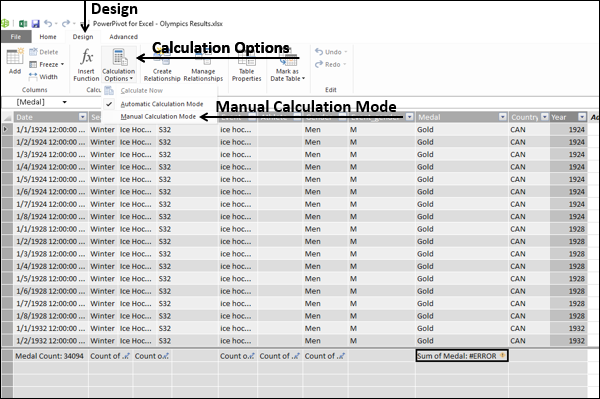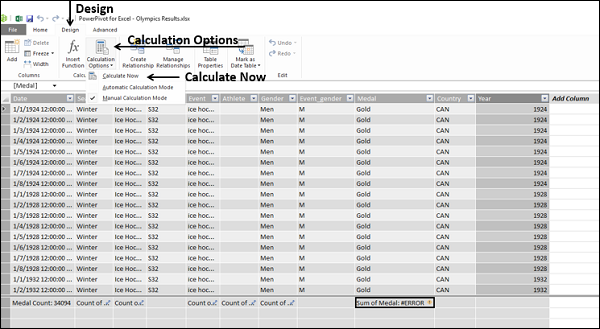# Excel DAX - Recalculating DAX Formulas

Recalculation of a DAX formula is required to reflect changes in the data and changes in the formula itself. However, recalculating a DAX formula involves performance cost.

Even then, to obtain accurate results, recalculation is essential. During recalculation, column dependencies are checked and you will be notified if a column has changed, if the data is invalid or if an error has appeared in a DAX formula that used to work.

## Types of Recalculation

You have two options for recalculating DAX formulas −

• Automatic Recalculation Mode (default)
• Manual Recalculation Mode

By default, Power Pivot automatically recalculates as required while optimizing the time required for processing. However, you can choose to update calculations manually, if you are working with complex formulas or very large data sets and want to control the timing of updates.

Both automatic and manual modes of recalculating DAX formulas have advantages. However, the recommended way is to use automatic recalculation mode. This way you can keep the Power Pivot data in sync and prevent problems caused by deletion of data, changes in names or data types or missing dependencies.

## Recalculating DAX Formulas Automatically

If you choose the default mode of recalculating DAX formulas, i.e. recalculating automatically, any changes to data that would cause the result of any DAX formula to change will trigger recalculation of the entire column that contains the DAX formula.

The following changes always require recalculation of DAX formulas −

• Values from an external data source have been refreshed.

• The DAX formula itself is changed.

• Names of tables or columns that are referenced in the DAX formula have been changed.

• Relationships between tables have been added, modified or deleted.

• New calculated fields or calculated columns have been added.

• Changes have been made to other DAX formulas within the workbook, so columns or calculations that depend on those DAX formulas need to be recalculated.

• Rows have been inserted or deleted in the table.

• You applied a filter that requires execution of a query to update the data set. The filter could have been applied either in a DAX formula or as part of a PivotTable or PivotChart.

## When to Use Manual Recalculation Mode?

You can use manual recalculation mode until you are ready with all your required DAX formulas in your workbook. This way, you can avoid incurring the cost of computing formula results on the workbook that is still in the draft state.

You can use manual recalculation of DAX formulas in the following conditions −

• You are designing a DAX formula by using a template and want to change the names of the columns and tables used in the DAX formula before you validate it.

• You know that some data in the workbook has changed but you are working with a different column that has not changed so you want to postpone a recalculation.

• You are working in a workbook that has many dependencies and want to defer recalculation till you are sure that all the necessary changes have been made.

But, you should be aware that as long as the workbook is configured to manual recalculation mode, any validation or checking of formulas is not performed. This will result in the following −

• Any new formulas that you add to the workbook will be flagged as containing an error.

## Configuring the Workbook for Manual Recalculation

As you have learnt, automatic recalculation is the default mode in the Data Model of any workbook. To configure a workbook for manual recalculation, do the following −

• Click the Design tab on the Ribbon in the Power Pivot window.
• Click the Calculation Options in the Calculations group.
• Click the Manual Calculation Mode in the dropdown list.## Recalculating DAX Formulas Manually

To recalculate the DAX formulas manually, do the following −

• Click the Design tab on the Ribbon in the Power Pivot window.
• Click the Calculation Options field in the Calculations group.
• Click the Calculate Now field in the dropdown list.